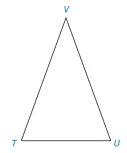Chapter 3.3, Problem 1E### Elementary Geometry for College St...

6th Edition
Daniel C. Alexander + 1 other
ISBN: 9781285195698

#### Solutions

Chapter
Section### Elementary Geometry for College St...

6th Edition
Daniel C. Alexander + 1 other
ISBN: 9781285195698
Textbook Problem
1 views

# For Exercises 1 to 8, use the accompanying drawing. If V U ¯ ≅ V T ¯ , what type of triangle is Δ V T U ?To determine

The nature of triangle ΔVTU.

Explanation

Definition:

If two sides of a triangle are congruent then it is called as isosceles triangle.

Given:

The given triangle is,

Figure (1)

And,

VU¯VT¯

Calculation:

Consider the triangle with congruent sides VU¯ and VT¯.

### Still sussing out bartleby?

Check out a sample textbook solution.

See a sample solution

#### The Solution to Your Study Problems

Bartleby provides explanations to thousands of textbook problems written by our experts, many with advanced degrees!

Get Started

#### Find more solutions based on key concepts